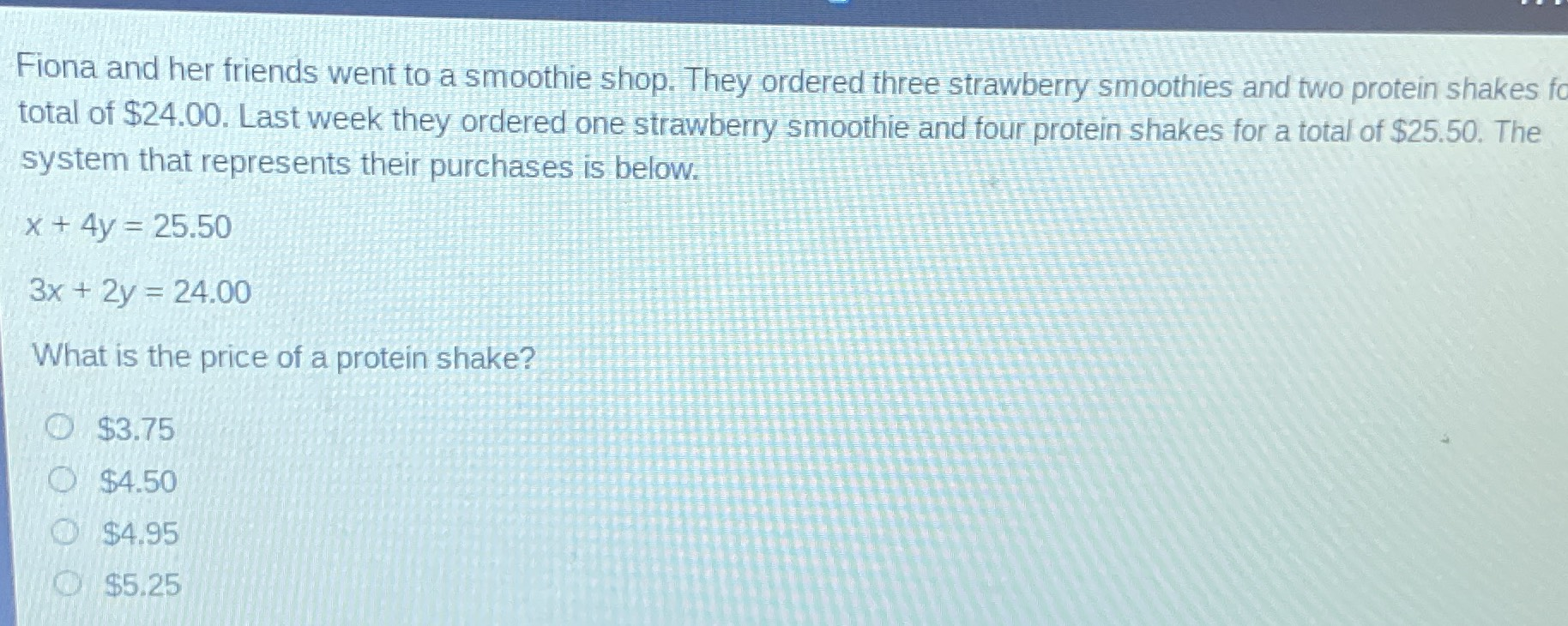### Still have math questions?

Algebra
QuestionFiona and her friends went to a smoothie shop. They ordered three strawberry smoothies and two protein shakes for total of $$\ 24.00$$ . Last week they ordered one strawberry smoothie and four protein shakes for a total of $$\ 25.50$$ . The system that represents their purchases is below.

$$x + 4 y = 25.50$$

$$3 x + 2 y = 24.00$$

What is the price of a protein shake?

A. $$\ 3.75$$

B. $$\ 4.50$$

C. $$\ 4.95$$

D. $$\ 5.25$$